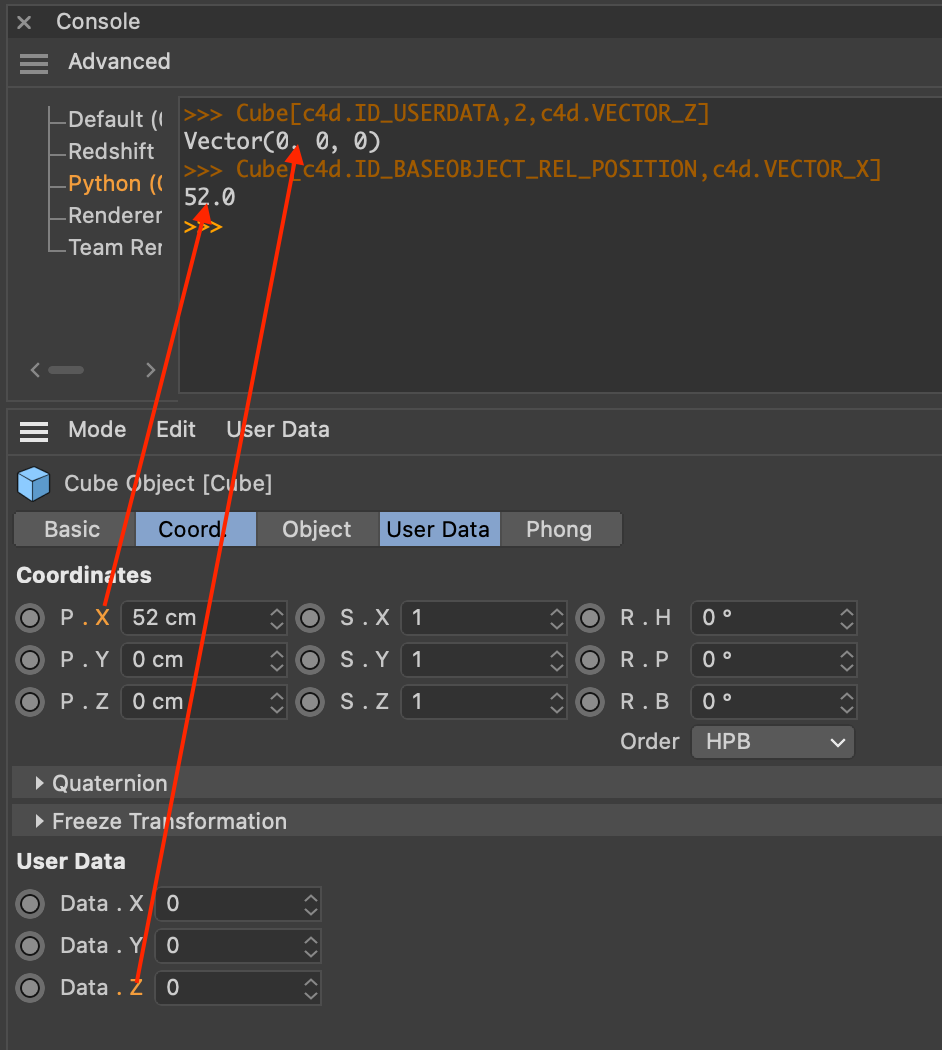# SOLVEDHow to get/set single vector value from USERDATA

Hello!
After dragging to console there is no way to get single value from vector UserData parameter.

``````>>> Cube[c4d.ID_USERDATA,2,c4d.VECTOR_Z]
Vector(0, 0, 0)
>>> Cube[c4d.ID_BASEOBJECT_REL_POSITION,c4d.VECTOR_X]
52.0
``````And no way to set this single value:

``````>>> Cube[c4d.ID_USERDATA,2,c4d.VECTOR_Z] = 12
Traceback (most recent call last):
File "console", line 1, in <module>
TypeError: __setitem__ expected c4d.Vector, not int
``````

There is a soution without creating a new c4d.Vector() variable?I need to get dragged to plugin dialog c4d.DRAGTYPE_DESCID from one single vector UserData parameter.
Thank you!

Hello @mikeudin,

Thank you for reaching out to us. Vectors represented by the type `c4d.Vector` are immutable in Cinema 4D, so one intentionally cannot change one of the components of a vector after it has been instantiated (matrices are not immutable over their vector components on the other hand). To achieve what you want to do, you must write:

``````old = Cube[c4d.ID_USERDATA, 2]
Cube[c4d.ID_USERDATA, 2] = c4d.Vector(old.x, old.y, 12)
``````

The following would also be possible, but it is not an improvement IMHO:

``````Cube[c4d.ID_USERDATA, 2] = c4d.Vector(Cube[c4d.ID_USERDATA, 2].x, Cube[c4d.ID_USERDATA, 2].y, 12)
``````

Cheers,
Ferdinand

Hello @mikeudin,

Thank you for reaching out to us. Vectors represented by the type `c4d.Vector` are immutable in Cinema 4D, so one intentionally cannot change one of the components of a vector after it has been instantiated (matrices are not immutable over their vector components on the other hand). To achieve what you want to do, you must write:

``````old = Cube[c4d.ID_USERDATA, 2]
Cube[c4d.ID_USERDATA, 2] = c4d.Vector(old.x, old.y, 12)
``````

The following would also be possible, but it is not an improvement IMHO:

``````Cube[c4d.ID_USERDATA, 2] = c4d.Vector(Cube[c4d.ID_USERDATA, 2].x, Cube[c4d.ID_USERDATA, 2].y, 12)
``````

Cheers,
Ferdinand